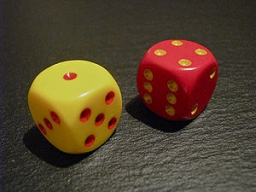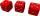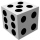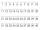# Two dice

We roll two dice. What is the probability that the sum of the falling numbers is greater than 3?

p =  0.9167

### Step-by-step explanation:Did you find an error or inaccuracy? Feel free to write us. Thank you!Tips to related online calculators
Would you like to compute count of combinations?

## Related math problems and questions:

• We rollWe roll two dice A. - what is the probability that the sum of the falling numbers is at most 4 B. - is at least 10 C. - is divisible by 5?
• Three diceWhat is the probability that when we roll three dice, we roll the numbers 1,2,3?
• Roll the diceWhat is the probability that if we roll the dice, a number less than 5 falls?
• One threeWe throw two dice. What is the probability that max one three falls?
• Sum or productWhat is the probability that two dice fall will have the sum 7 or product 12?
• ThrowWe throw 2 times with 2 dices. What is the probability that the first roll will fall more than sum of 9 and the second throw have sum 3 or does not have the sum 4?
• The diceWhat is the probability of events that if we throw a dice is rolled less than 6?
• Fall sum or sameFind the probability that if you roll two dice, it will fall the sum of 10, or the same number will fall on both dice.
• Sum on diceWe have two dice. What is the greater likelihood of fall a total sum 7 or 8 ? (write 7, 8 or 0 if the probabilities are the same)?
• DiceWe throw five times the dice. What is the probability that six fits exactly twice?
• Three diceThe player throwing the three dice asked G. Galilei the question: "Should I bet on the sum of 11 or the sum of 12?" What did Galilei answer him? Hint: write down all three triples of numbers that can be thrown and: have a total of 11 have a total of 12 an
• Dice and coinA number cube is rolled and a coin is tossed. The number cube and the coin are fair. What is the probability that the number rolled is greater than 2 and the coin toss is head?
• Three-digit numbersWe have digits 0,1,4,7 that cannot be repeated. How many three-digit numbers can we write from them? You can help by listing all the numbers.
• DicesWe will throw two dice. What is the probability that the ratio between numbers on first and second dice will be 1:2?
• One diceCalculate the probability of one dice roll with the numbers 1, 2, 3, 4, 5, 6 on the walls. Write the results in a notebook in the shape of a fraction in the basic form: 2/3. a, The number 1 falls on the cube. b, The number 5 falls on the cube. c, An even
• Six on diceWhat is the probability that when throwing two dice will fall at least one six?
• How manyHow many double-digit numbers greater than 30 we can create from digits 0, 1, 2, 3, 4, 5? Numbers cannot be repeated in a two-digit number.